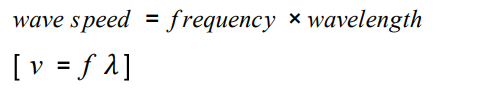# Properties of Waves Knowledge questions for AQA GCSE Physics and Combined Science

1. Sketch a transverse wave and label the amplitude, crest and trough. Also, label the wavelength of the wave in two places.
2. Draw a line to represent the undisturbed position of the wave and label it clearly.
3. Copy these three important definitions.
1. The amplitude of a wave is the maximum displacement of a point on a wave away from its undisturbed position.
2. The wavelength of a wave is the distance from a point on one wave to the equivalent point on the adjacent wave.
3. The frequency of a wave is the number of waves passing a point each second.
4. What is meant by the period of a wave?
5. Write down an equation in terms of the period that can be used to work out the frequency of a wave.
6. What are units of frequency?
7. What are the units of the period of a wave – tick-tock?
8. Copy the following definition of a wave. The wave speed is the speed at which the energy is transferred (or the wave moves) through the medium.
9. Consider this equation:10. Can you rearrange it so that speed, frequency and wavelength are all the subject of the equation? E.g. frequency =, wavelength =
11. Describe a method to measure the speed of sound waves in air.
12. Describe a method to measure the speed of ripples on a water surface.

Physics Only

1. What happens to the speed of sound waves when they enter a denser medium?  E.g from air into water.
2. What happens to the frequency of sound waves when they enter a denser medium?
3. What happens to the wavelength of sound waves when they enter a denser medium?